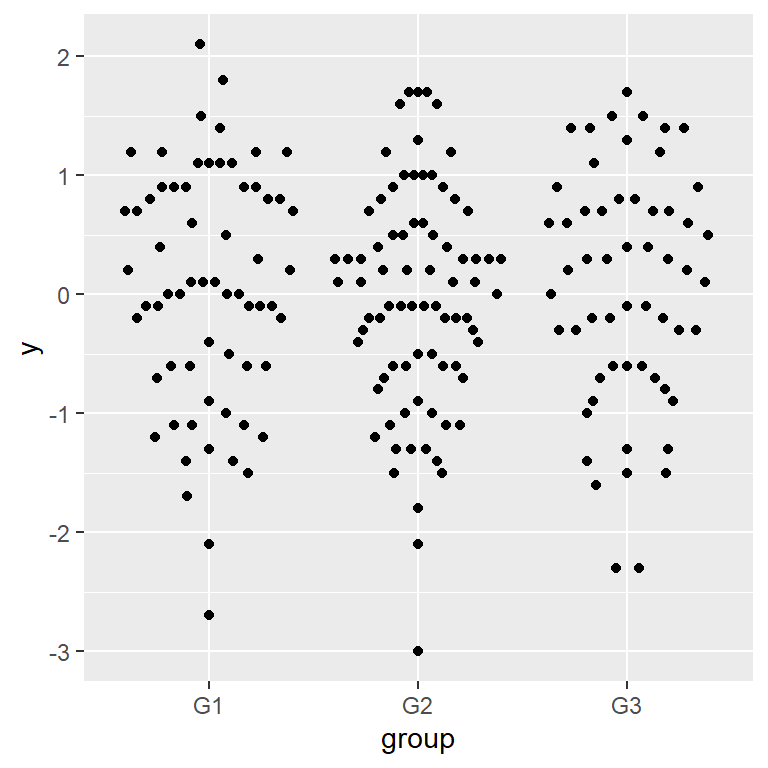# Beeswarm in ggplot2 with ggbeeswarm

Erik Clarke

## Sample data

The following data frame contains the measure of a variable within three different groups. We will use this data in the examples below.

``````# Sample data
set.seed(1995)
y <- round(rnorm(200), 1)

df <- data.frame(y = y,
group = sample(c("G1", "G2", "G3"),
size = 200,
replace = TRUE))``````

## Beeswarm plot in ggplot2 with `geom_beeswarm`

The `ggbeeswarm` package contains a function named `geom_beeswarm`, which can be used to create a beeswarm in ggplot2.

``````# install.packages("ggplot2")
library(ggplot2)
# install.packages("ggbeeswarm")
library(ggbeeswarm)

# Basic beeswarm plot in ggplot2
ggplot(df, aes(x = group, y = y)) +
geom_beeswarm()``````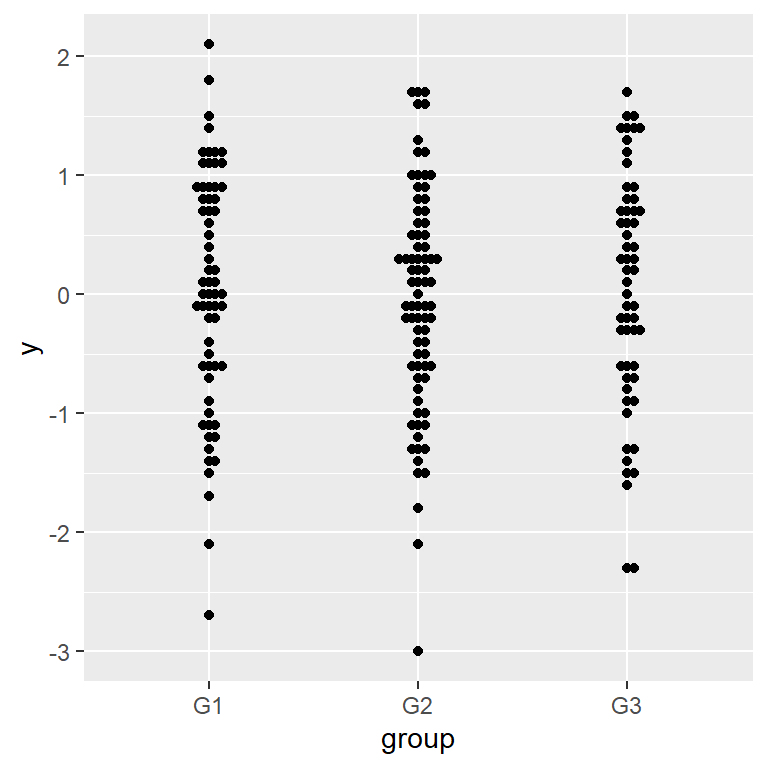Size and scaling

By default the observations are shown all very close to each other. You can use the `cex` argument to increase the spacing and `size` to increase the size of the point.

``````# install.packages("ggplot2")
library(ggplot2)
# install.packages("ggbeeswarm")
library(ggbeeswarm)

# Beeswarm plot in ggplot2
ggplot(df, aes(x = group, y = y)) +
geom_beeswarm(cex = 3)``````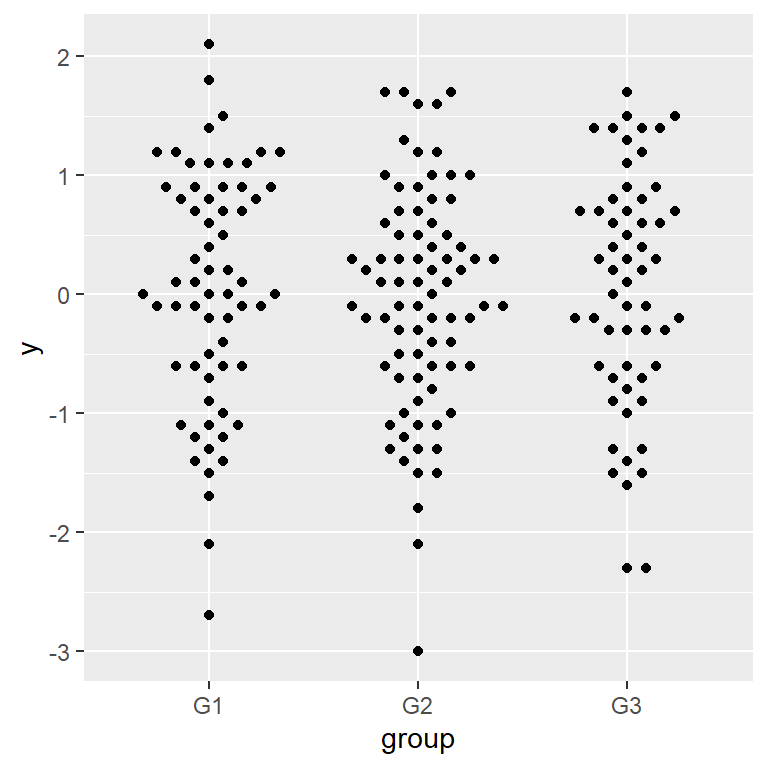Color by group

As with other ggplot2 charts if you want to color the observations by group you can pass the categorical variable to the `color` or `colour` argument of `aes`.

``````# install.packages("ggplot2")
library(ggplot2)
# install.packages("ggbeeswarm")
library(ggbeeswarm)

# Beeswarm plot in ggplot2
ggplot(df, aes(x = group, y = y, color = group)) +
geom_beeswarm(cex = 3)``````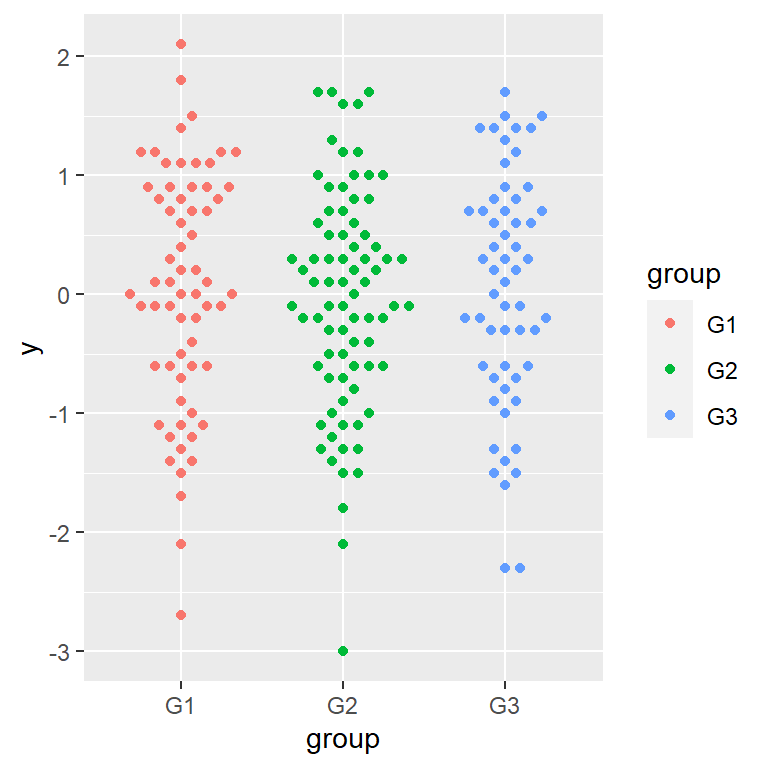Change the colors

If you color the observations by group a default color scale will be used. You can change the colors with `scale_color_brewer`, `scale_color_manual` or an equivalent color scale.

``````# install.packages("ggplot2")
library(ggplot2)
# install.packages("ggbeeswarm")
library(ggbeeswarm)

# Beeswarm plot in ggplot2
ggplot(df, aes(x = group, y = y, color = group)) +
geom_beeswarm(cex = 3) +
scale_color_brewer(palette = "Set1")``````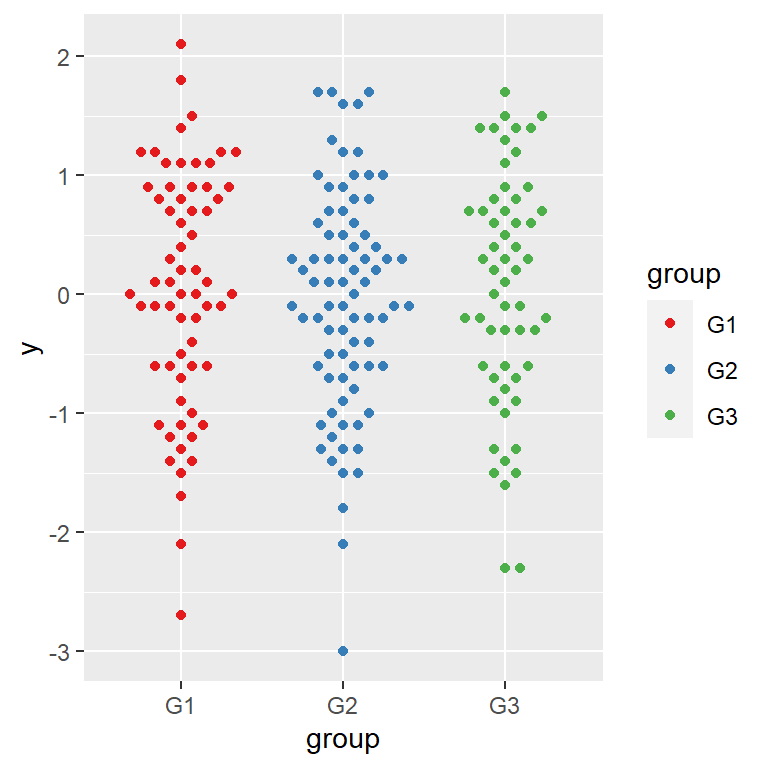Priority methods

In order to perform point layout a priority method must be chosen. By default the ascending method is used but you can change it with the `priority` argument. The other possible values are `"descending"`, `"density"`, `"random"` and `"none"`.

``````# install.packages("ggplot2")
library(ggplot2)
# install.packages("ggbeeswarm")
library(ggbeeswarm)

# Beeswarm plot in ggplot2
ggplot(df, aes(x = group, y = y, color = group)) +
geom_beeswarm(cex = 3,
priority = "density")``````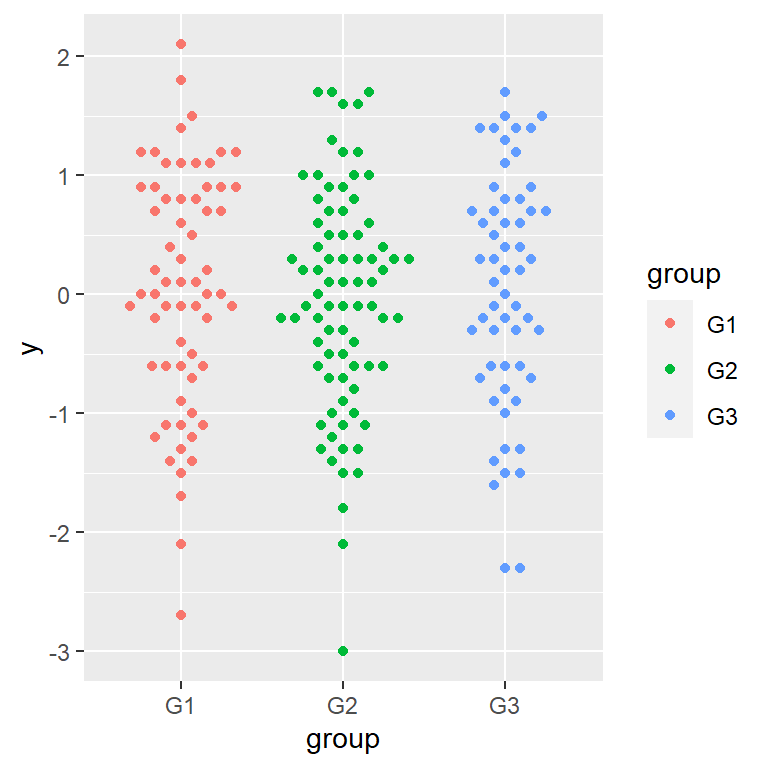## Adding quasi-random noise with `geom_quasirandom`

The `ggbeeswarm` package also contains `geom_quasirandom`, which draws jittered data points similarly to `geom_jitter` but reducing overplotting using a van der Corput sequence or Tukey texturing.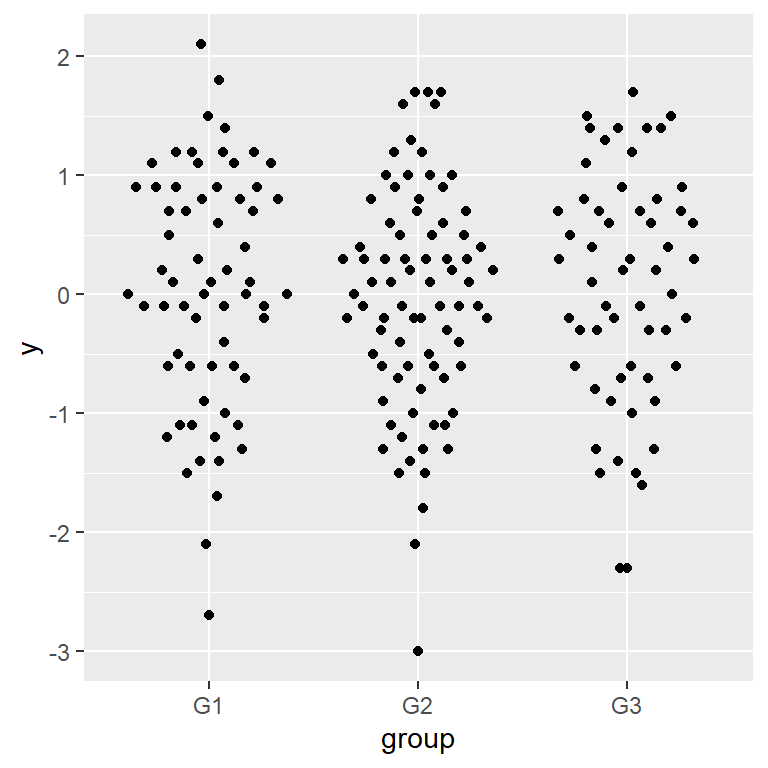In order to add jittered data points without overplotting use the `geom_quasirandom` function as shown below.

``````# install.packages("ggplot2")
library(ggplot2)
# install.packages("ggbeeswarm")
library(ggbeeswarm)

# van der Corput sequence or Tukey texturing
ggplot(df, aes(x = group, y = y)) +
geom_quasirandom()``````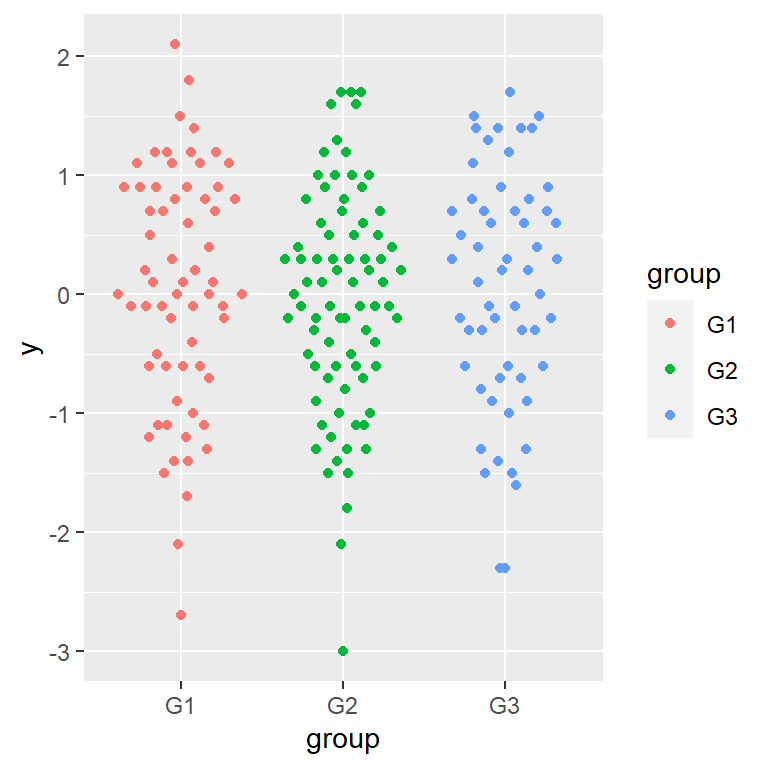Color by group

As with `geom_beeswarm` you can color the observations by group if needed, passing the categorical variable to `color`.

``````# install.packages("ggplot2")
library(ggplot2)
# install.packages("ggbeeswarm")
library(ggbeeswarm)

# van der Corput sequence or Tukey texturing
ggplot(df, aes(x = group, y = y, color = group)) +
geom_quasirandom()``````

### Methods

There are different methods to distribute the points. By default the `"quasirandom"` method is used but you can also set `"pseudorandom"`, `"smiley"` or `"frowney"`.

“pseudorandom” method

``````# install.packages("ggplot2")
library(ggplot2)
# install.packages("ggbeeswarm")
library(ggbeeswarm)

# pseudorandom method
ggplot(df, aes(x = group, y = y)) +
geom_quasirandom(method = "pseudorandom")``````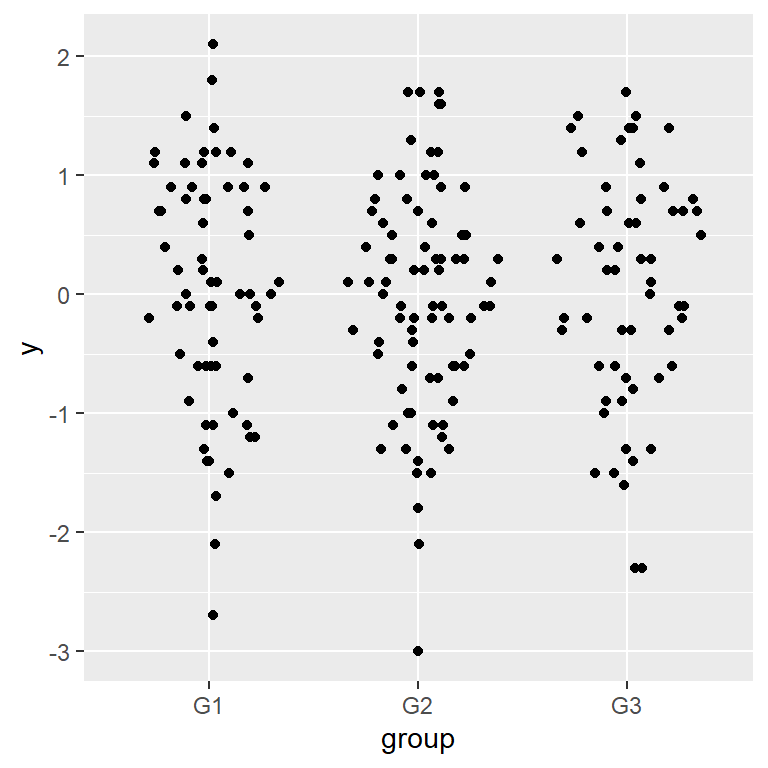“smiley” method

``````# install.packages("ggplot2")
library(ggplot2)
# install.packages("ggbeeswarm")
library(ggbeeswarm)

# smiley method
ggplot(df, aes(x = group, y = y)) +
geom_quasirandom(method = "smiley")``````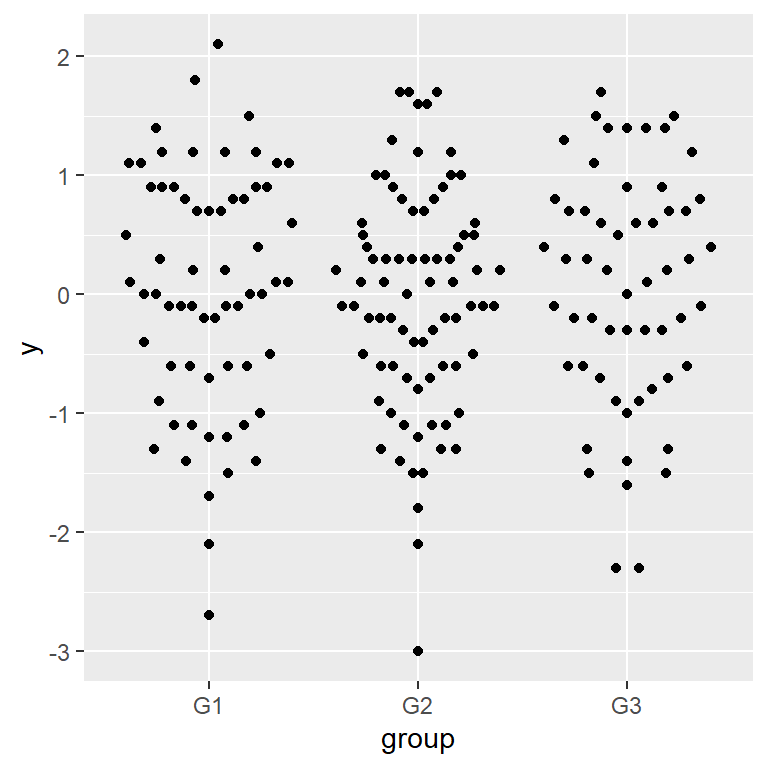“frowney” method

``````# install.packages("ggplot2")
library(ggplot2)
# install.packages("ggbeeswarm")
library(ggbeeswarm)

# frowney method
ggplot(df, aes(x = group, y = y)) +
geom_quasirandom(method = "frowney")``````